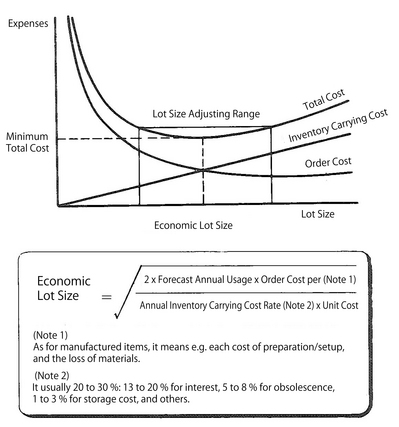#### Asprova MRP

Asprova MRP is calculated on the memory requirement, allocate lead time at a high speed. Can achieve material arrangement Just in Time, which is impossible in other MRP system.

MRP glossary TOP > E > Economic Lot Size

## Economic Lot Size

Economic Lot Size,EOQ,Economic Order Quantity

Only necessary quantity of items are not always ordered (instructed for manufactured items) for each necessary item. In most cases, the order quantity (manufacturing quantity for manufactured items) is determined by the balance between the order cost (the cost for preparation such as logistics for manufactured items) for the necessary items and the inventory carrying cost. This order quantity is generally called lot or lot size. Economic Lot Size (ELS for short) refers to the best lot quantity to make the total cost minimum by considering the balance between ordering cost and inventory carrying cost, which are contradictory. As shown in the figure, for example, the larger the lot size is, the higher the average inventory level is and its inventory carrying cost also increase by a certain ratio. But the ordering cost a unit decreases because the transportation and logistics costs are allocated to many items. On the other hand, the smaller the lot size is, the lower the average inventory level is. However, on the contrary, the ordering cost a unit increases. When the total cost of ordering cost and inventory carrying cost is minimum, the lot size is the most economic. Economic Lot Size is also called EOQ (standing for Economic Order Quantity) and is calculated by means of the following formula:Related term: Lot Sizing

Reference：JIT Business Research Mr. Hirano Hiroyuki